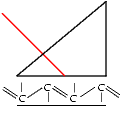# RSim     Single layer antireflection coating

Again we are in the wavelength regime.
No we simulate a single layer quarter wave coating designed for a single wavelength.
For a perfect antireflection coating the film index has to be n1 = sqrt(n0*n7) and the optical thickness n1*d1 = lambda/4. A low index glas lens (n7=1.5151) for example demands then a coating with a index of n1= 1.2309 when immersed in air, which is not available. So in the real world the reflected intensity will not drop to 0% for the given wavelength and this glas type.
In the example I choose n1= 1.38 ( MgF2 ) , which gives d1= 99.637 nm for lambda = 550 nm. Set the scaling from 0 to 0.04 ( the amount of reflected light without a coating at theta = 0 degree) and see the effect of the coating. Increase the substrat index n7 and watch the reflectivity decrease.